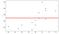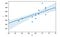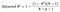# R-squared statistic : basic intuition

The quality of a regression model is typically accessed using two related quantities: Residual Standard Error (RSE) and the R squared statistic. The RSE provides an absolute measure of lack of fit of the model to the data using the following formula-

However, RSE is measured in the units of the dependent variable (Y) and it is not always clear what constitutes a good RSE. Alternatively, R squared is a statistical measure that tells us how well the data fit in a regression model. It measures the proportion of variance in the dependent variable (Y) that can be explained by the independent variable (X). It can any value between 0 to 1 and is independent of the scale of Y. The formula for R squared is -

or simply, we can write as-

where, TSS is the total sum of squares and RSS is the residual sum of squares. TSS measures the total variance in the response Y and can be defined as the amount of variability inherent in the response before the regression is performed. On the other way, RSS measures the amount of variability that is left unexplained after performing regression. Therefore, (TSS-RSS) measures the amount of variability in the response that is explained (or removed) by performing the regression, and R squared measures the proportion of variability in Y that can be explained using X. Let’s take a small data set as follows-Scatter plot of the data set, where red line depicts the mean of Y.

If we take the mean of all the points of Y, we will get it as 187.71. Then, TSS will be as follows -

So without least-squares regression, our sum of squares is 9328.85. It is also visible that the red line doesn’t seem to fit the data very well.

Again, if we plot the regression line for the above data set, then we will get it as-Regression line

The above regression line seems to fit the data pretty well, but to measure how much better it fits, we have to measure the sum of squared residuals, i.e. RSS which is found to be 4382.89.

Without using regression, our model had an overall sum of squares of 9328.85. Using least-squares, regression reduced that down to 4382.89. So using least-squares, regression eliminated a considerable amount of prediction error.

So, the total reduction is (9328.85–4382.89) = 4945.96

We can represent this reduction as a percentage of the original amount of prediction error:

((9328.85–4382.89)/(9328.85))*100 = 0.5301 * 100 = 53.01%

Here, R-squared = 0.5301 ,i.e. almost half of the variability in Y is explained by a linear regression on X.

So, R-squared tells us what percent of the prediction error in the dependent variable, Y is eliminated when we use least-squares regression on the independent variable, X.

An R-Squared statistic that is close to 1 indicates that a large proportion of the variability in the response has been explained by the regression. A number near 0 indicates that the regression did not explain much of the variability in the response.

# Adjusted R-Squared :

If we add some predictors to the model, R-squared tends to increase irrespective of the usefulness of the predictors. As R-Squared always increases and never decreases, it can appear to be a better fit with the more variables we add to the model. This can be completely misleading. Adjusted R-squared tells us the proportion of variation explained by only the independent variables that actually affect the dependent variable. The adjusted R-Squared will penalize for adding independent variables that do not fit the model. Adjusted R-squared will always be less than or equal to R-squared. The formula for adjusted R-Squared is :where, N = the number of points in the data sample and p = the number of independent variables in the model, excluding the constant.

## Geek Culture

Proud to geek out. Follow to join our +500K monthly readers.

## Geek Culture

A new tech publication by Start it up (https://medium.com/swlh).

Written by

## Dhrubjun

Machine learning/Data Science enthusiast## Geek Culture

A new tech publication by Start it up (https://medium.com/swlh).

## More From Medium

Medium is an open platform where 170 million readers come to find insightful and dynamic thinking. Here, expert and undiscovered voices alike dive into the heart of any topic and bring new ideas to the surface. Learn more

Follow the writers, publications, and topics that matter to you, and you’ll see them on your homepage and in your inbox. Explore

If you have a story to tell, knowledge to share, or a perspective to offer — welcome home. It’s easy and free to post your thinking on any topic. Write on Medium